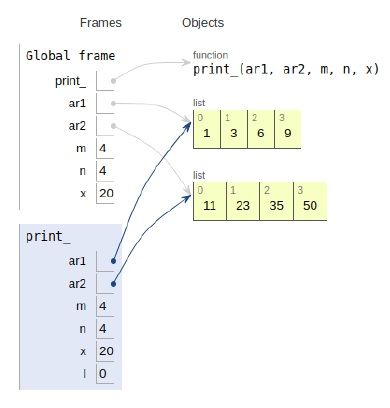# Python Program for Find the closest pair from two sorted arrays

Problem statement − We are given two arrays, we need to find the closest pair from the two sorted arrays

Now let’s observe the solution in the implementation below −

## Example

Live Demo

# sys module
import sys
# pair
def print_(ar1, ar2, m, n, x):
# difference
diff=sys.maxsize
# index
l = 0
r = n-1
while(l < m and r >= 0):
# closest pair
if abs(ar1[l] + ar2[r] - x) < diff:
res_l = l
res_r = r
diff = abs(ar1[l] + ar2[r] - x)
# pair sum
if ar1[l] + ar2[r] > x:
r=r-1
else:
l=l+1
# Print the result
print("The closest pair available is [",ar1[res_l],",",ar2[res_r],"]")
# main
ar1 = [1, 3, 6, 9]
ar2 = [11, 23, 35, 50]
m = len(ar1)
n = len(ar2)
x = 20
print_(ar1, ar2, m, n, x)

## Output

The closest pair available is [ 9 , 11 ]All the variables are declared in the local scope and their references are seen in the figure above.

## Conclusion

In this article, we have learned about how we can make a Python Program for Find the closest pair from two sorted arrays